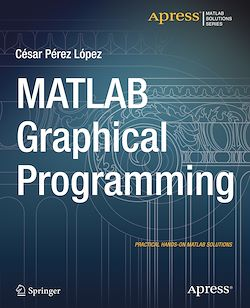# MATLAB Graphical Programming

-English
208 Pages

Description

MATLAB enables you to work with its graphics capabilities in almost all areas of the experimental sciences and engineering. The commands that MATLAB implements in job related graphics are quite useful and are very efficient.

MATLAB has functions for working with two-dimensional and three-dimensional graphics, statistical graphs, curves and surfaces in explicit, implicit, parametric and polar coordinates. It also works perfectly with twisted curves, surfaces, volumes and graphical interpolation. MATLAB Graphical Programming addresses all these issues by developing the following topics:This book is a reference designed to give you a simple syntax example of the commands and to graph it so that you can see the result for:

Subjects

##### Software development

Informations